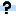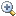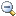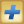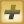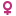Marilyn =HEB(Miriyam מרים)=YID(Mirl,Mariem מאריעם,מירל)=MIDGER(Merlen)=ROM(Marica,Mina)=RUS(Mara,Marina) “Molly” Feldmann (Feldman) {=Feldmann's __th generation}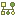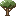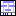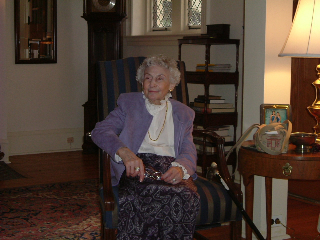i1 OR827bOR189fe 2009-04-19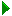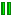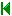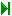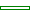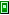Marilyn, known as Molly, was born in 1923 in Philadelphia.1
• Birth Notes
Molly's father was Samuel =HEB(Shmuel שמואל)=YID(Schmelke,Schmil,Zenwil שמיל,שמלקא,זנוויל)=ROM(Samoil,Samuel)=ENG(Samuel,Sam)=GER(Sanwel) Feldmann (Feldman) {=Feldmann's __th generation} and her mother was Elizabeth (born Leia) =HEB(Leah לאה)=YID(Leie לאה)=ROM(Leia,Lia) “Liva” Finkelstein {=Finkelstein's __th generation}.  Her paternal grandparents were Efrayim =HEB(Efrayim אפריים)=YID(Froim,Fischl פרוים,פישל)=ROM(Froiche,Fredi)=ENG(Fred)=GER(Ferdinand,Frederik,Fritz)=RUS(Fima) Feldmann (Feldman) {=Feldmann's __th generation} and MISSING GIVENAME MISSING SURNAME {=__th generation}; her maternal grandparents were Moishe =HEB(Mosheh משה)=YID(Moische משה)=ROM(Moişe,Moise,Moriţ,Moscu,Moşcu,Mozes)=ENG(Moses,Morris)=GER(Moritz) Finkelstein {=Finkelstein's __th generation} and Molke =HEB(Malkah מלכה)=YID(Molke מלכה)=ROM(Malca,Amalia)=POL(Regina) Kohen (Cohn) {=KOHEN's __th generation}. She had a sister named Efrayim.2

• Personal Timeline

•